• 用matlab画小车运动轨迹
2021-04-19 08:51:05

基于无碳小车前轮运动关系的MATLAB运动轨迹仿真

基于无碳小车前轮运动关系的MATLAB运动轨迹仿真

clear all;clc;

R=50;

r=15;

L=150;

k=1/5;

e=12.5;

arf0=0;

fx1=@(sita)(cos(arf0-R*r*r/k/L/e/e*sin(sita)-atan(r*cos(sita)/e))*R/k./cos(atan(r*cos(sita)/e)));

fx2=@(sita)(sin(arf0-R*r*r/k/L/e/e*sin(sita)-atan(r*cos(sita)/e))*R/k./cos(atan(r*cos(sita)/e)));

sita=0:0.05:4*pi;

for ii=1:length(sita)

sita0=sita(ii);

end

plot(x,y);

基于无碳小车转弯半径计算方法的MATLAB运算

clc;clear all;

a=100;

b=50;

L=150;

sita=0.9;

arf=0.9;

B=100;

A=a*cos(sita)/b;

beta=atan(sin(arf)/(A+cos(arf)));

Rg=b/sin(beta)

Rq=Rg*cos(beta)/cos(sita)

Of=sqrt(Rg*Rg+b*b-2*Rg*b*sin(beta))

Rw=sqrt(Of*Of+B*B/4+B*Of)

clc;clear all;

a=100;

b=50;

L=150;

sita=-0.9:0.01:0.9;

arf=sita;

B=100;

A=a*cos(sita)/b;

beta=atan(sin(arf)./(A+cos(arf)));

Rg=b./sin(beta);

Rq=Rg.*cos(beta)./cos(sita);

Of=sqrt(Rg.*Rg+b*b-2*Rg*b.*sin(beta));

Rw=sqrt(Of.*Of+B*B/4+B*Of);

plot(sita,Rg,sita,Rq,sita,Of,sita,Rw);

axis(-0.9,0.9)

更多相关内容
• ## MATLAB画小车

千次阅读 2022-04-14 22:08:47
MATLAB画小车 这里参考了robotarium库的代码 具体实现如下： my_gritsbot_patch函数，完成小车的图形绘制，可修改相关参数完成小车样式改变。 function [ patch_data ] = my_gritsbot_patch() %GRITSBOT_PATCH This...

## MATLAB画小车这里参考了robotarium库的代码

## 具体实现如下：

my_gritsbot_patch函数，完成小车的图形绘制，可修改相关参数完成小车样式改变。

function [ patch_data ] = my_gritsbot_patch()
%GRITSBOT_PATCH This is a helper function to generate patches for the
%simulated GRITSbots.  YOU SHOULD NEVER HAVE TO USE THIS FUNCTION.
%
% PATCH_DATA = GRITSBOT_PATCH() generates a struct containing patch data
% for a robot patch.

% Make it facing 0 rads
robot_width = 1;
robot_height = 2;
wheel_width = 0.2;
wheel_height = 0.4;
led_size = 0.1;

% Helper functions to generate vertex coordinates for a centered
% rectangle and a helper function to shift a rectangle.
rectangle = @(w, h) [w/2 h/2 1; -w/2 h/2 1; -w/2 -h/2 1; w/2 -h/2 1];
shift = @(r, x, y) r + repmat([x, y, 0], size(r, 1), 1);

% Create vertices for body, wheel, and led.
body = rectangle(robot_width, robot_height);
wheel = rectangle(wheel_width, wheel_height);
led = rectangle(led_size, led_size);

% Use pre-generated vertices and shift them around to create a robot
left_wheel_1 = shift(wheel, -(robot_width + wheel_width)/2, -robot_height/3);
right_wheel_1 = shift(wheel, (robot_width + wheel_width)/2, -robot_height/3);
left_wheel_2 = shift(wheel, -(robot_width + wheel_width)/2, robot_height/3);
right_wheel_2 = shift(wheel, (robot_width + wheel_width)/2, robot_height/3);
left_led = shift(led,  robot_width/4, robot_height/2 - 2*led_size);
right_led = shift(led,  -robot_width/4, robot_height/2 - 2*led_size);

% Putting all the robot vertices together
vertices = [
body ;
left_wheel_1;
left_wheel_2;
right_wheel_1;
right_wheel_2;
left_led;
right_led
];

% Only color the body of the robot.  Everything else is black.
colors = [
[255, 0, 0]/255;
0 0 0;
0 0 0;
0 0 0;
0 0 0;
1 1 1;
1 1 1
];

% This seems weird, but it basically tells the patch function which
% vertices to connect.
faces = repmat([1 2 3 4 1], 7, 1);

for i = 2:7
faces(i, :) = faces(i, :) + (i-1)*4;
end

patch_data = [];
patch_data.vertices = vertices;
patch_data.colors = colors;
patch_data.faces = faces;
end



主函数：


axis([-1,1,-1,1]);
data = my_gritsbot_patch;
this.robot_body = data.vertices;
x  = 0;
y  = 0;
th = 0;
rotation_matrix = [
cos(th) -sin(th) x;
sin(th)  cos(th) y;
0 0 1];
transformed = this.robot_body*rotation_matrix';
this.robot_handle{1} = patch(...
'Vertices', transformed(:, 1:2), ...
'Faces', data.faces, ...
'FaceColor', 'flat', ...
'FaceVertexCData', data.colors, ...
'EdgeColor','none');


小车运动请参考：MATLAB 轮式机器人轨迹跟踪仿真
轨迹跟踪代码：



clear all;clc;

%% define constant
l=0.2;
R=1;
K=0.1;
delta_T=0.5;

%% define initialization
Pos=[0,12,3/2*pi]; %X,Y,Theta
T=linspace(0,2*pi,200);
desire_Pos=[16*power(sin(T),3);13*cos(T)-5*cos(2*T)-2*cos(3*T)-cos(4*T)];

%%  Graphics
f3=figure;
xlabel('x (m)')
ylabel('y (m)')
grid on

%%  robot dimensions
data = my_gritsbot_patch;
this.robot_body = data.vertices;
x  = Pos(1);
y  = Pos(2);
th = Pos(3)-pi/2;
rotation_matrix = [
cos(th) -sin(th) x;
sin(th)  cos(th) y;
0 0 1];
transformed = this.robot_body*rotation_matrix';
this.robot_handle{1} = patch(...
'Vertices', transformed(:, 1:2), ...
'Faces', data.faces, ...
'FaceColor', 'flat', ...
'FaceVertexCData', data.colors, ...
'EdgeColor','none');

%% define controller

%% draw picture
h= animatedline('color','r','LineStyle','--');
h_car= animatedline('color','b');
h_car_model = animatedline('Marker','o','MaximumNumPoints',1);     %只显示1个新的点
axis([-20 20 -20 15])

for k = 1:length(T)

e_x=Pos(1)-desire_Pos(1,k);
e_y=Pos(2)-desire_Pos(2,k);
u_x=-K*e_x;
u_y=-K*e_y;
A_mat=[cos(Pos(3)) -l*sin(Pos(3));sin(Pos(3)) l*cos(Pos(3))];
P=[cos(Pos(3)) 0;sin(Pos(3)) 0;0 1];
U_mat=[u_x,u_y]';
v_w_mat=A_mat\U_mat;
Pos(1)=Pos(1)+v_w_mat(1)*cos(Pos(3))-v_w_mat(2)*l*sin(Pos(3));
Pos(2)=Pos(2)+v_w_mat(1)*sin(Pos(3))+v_w_mat(2)*l*cos(Pos(3));

Pos(3)=Pos(3)+v_w_mat(2)*delta_T;

x  = Pos(1);
y  = Pos(2);
th = Pos(3)-pi/2;
rotation_matrix = [
cos(th) -sin(th) x;
sin(th)  cos(th) y;
0 0 1];
transformed = this.robot_body*rotation_matrix';
set(this.robot_handle{1}, 'Vertices', transformed(:, 1:2));

frame=getframe(gcf);
im = frame2im(frame);
[imind,cm] = rgb2ind(im,256);
if k==1
imwrite(imind,cm,'experiment.gif','gif', 'LoopCount',inf,'DelayTime',0.000001);
end
if rem(k,2)==0
imwrite(imind,cm,'experiment.gif','gif','WriteMode','append','DelayTime',0.000001);
end
drawnow
end


展开全文目标跟踪
•    当我们仅仅使用MATLAB以可视化图像的形式去观察Gazebo中小车运动轨迹，并不参与小车的控制时，完全没有必要以栅格地图的形式去复现gazebo中的仿真环境，当环境较复杂或需要多变的时候往往需要很大的工作量，...

虽然，Gazebo中自带了绘图工具，当需要绘制一些简单的图像时，非常的方便，但是当需要绘制复杂的图像时，还是MATLAB更加合适一些。
当我们仅仅使用MATLAB以可视化图像的形式去观察Gazebo中小车的运动轨迹，并不参与小车的控制时，完全没有必要以栅格地图的形式去复现gazebo中的仿真环境，当环境较复杂或需要多变的时候往往需要很大的工作量，本文通过图片的形式，将gazebo中仿真环境
本文介绍一种通过图片的形式，将gazebo中仿真环境快速复现到MATLAB中，并在此基础上叠加显示仿真小车运动轨迹的方法

一、计算两幅地图间的变换比例

（1）首先确定gazebo中仿真环境的长度及宽度，先通过工具栏中的工具，将视野调至俯视图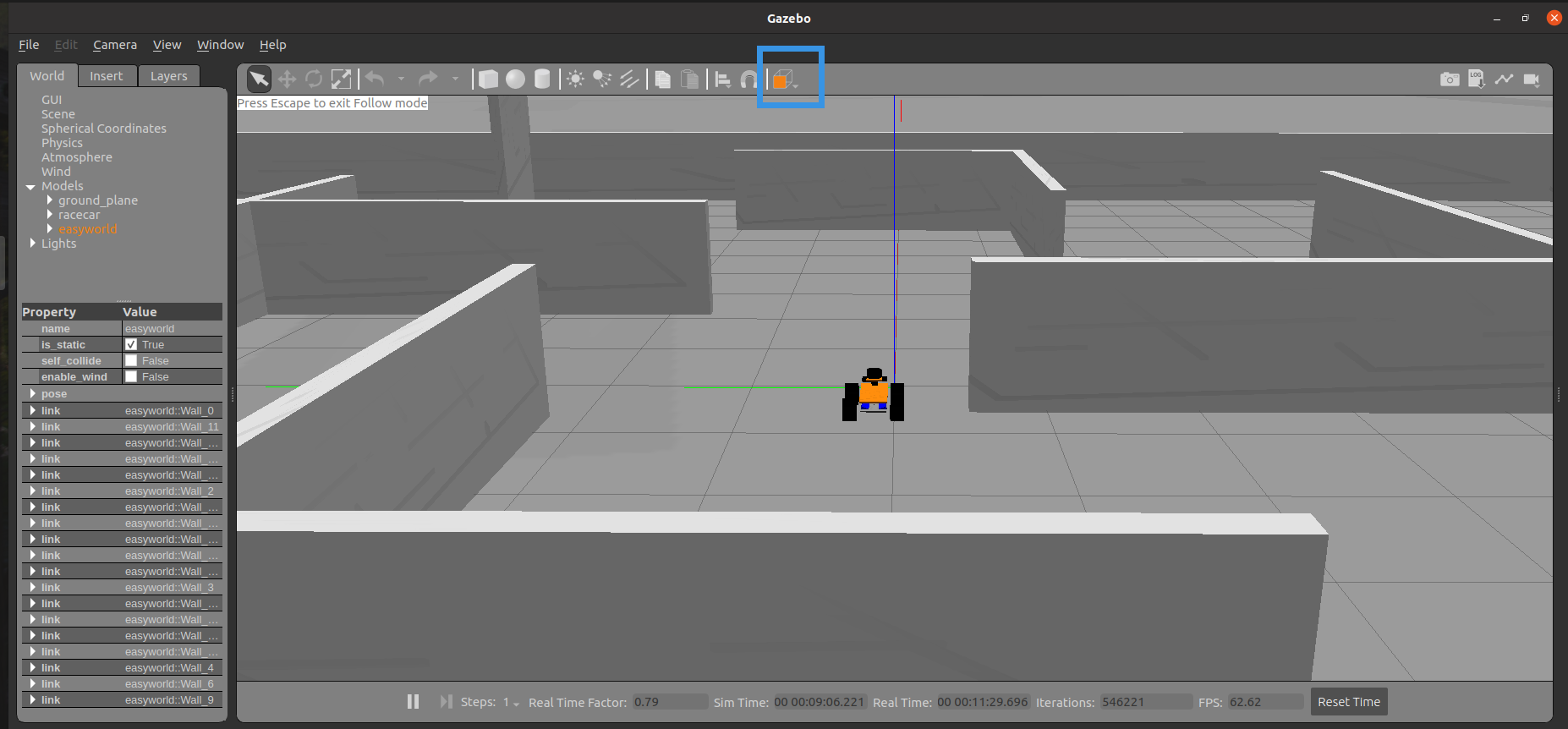依次选中gazebo的仿真环境的上下左右边界，并在左侧的pose栏中查看其坐标值，比如本文所用的例子中上边界为的X值为9.925000，下边界为-9.925，即在gazebo的仿真环境中，其在X轴上长度为19.85m。左边界的Y值为10.1235，右边界的Y值为-10.2265，即仿真环境在Y轴上长度为20.35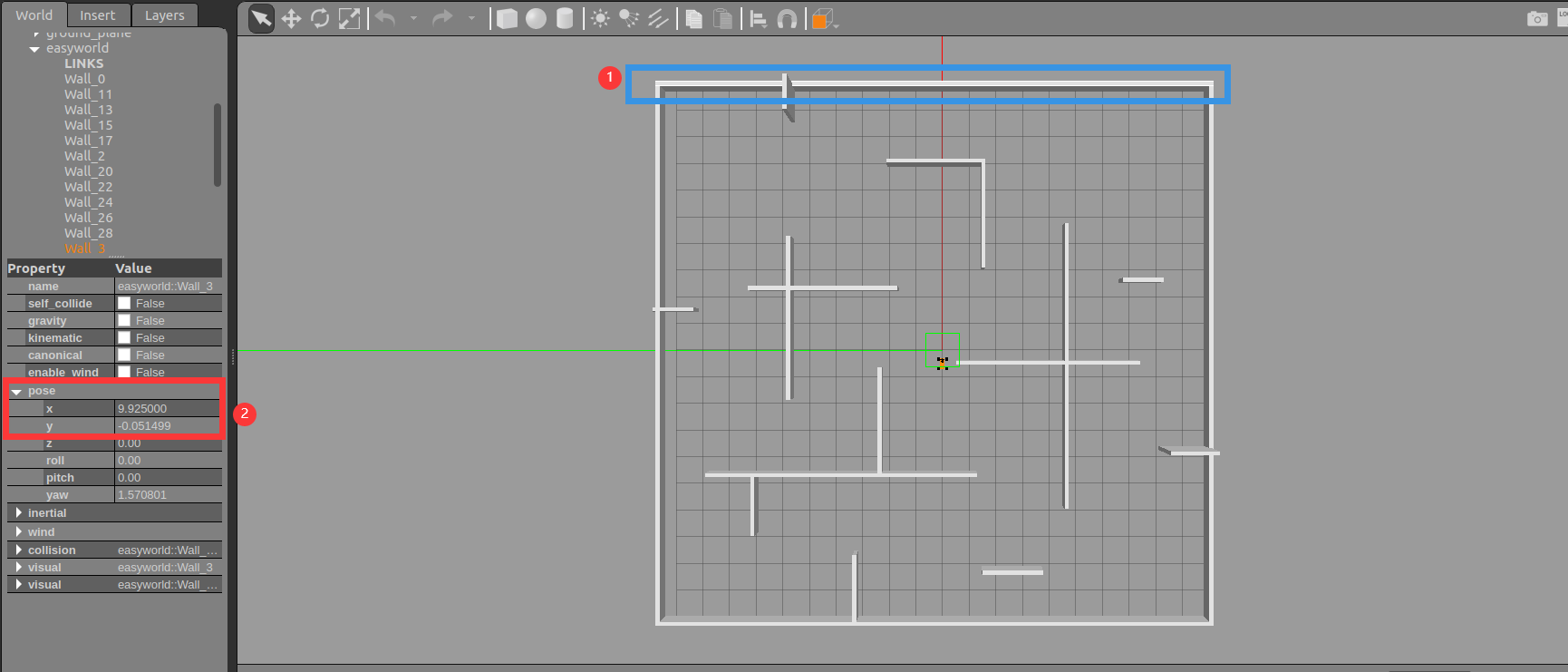（2）将俯视图下的仿真环境，截图并保存，作为MATLAB中的地图使用，截图前，可在view菜单栏，取消Origin坐标轴的显示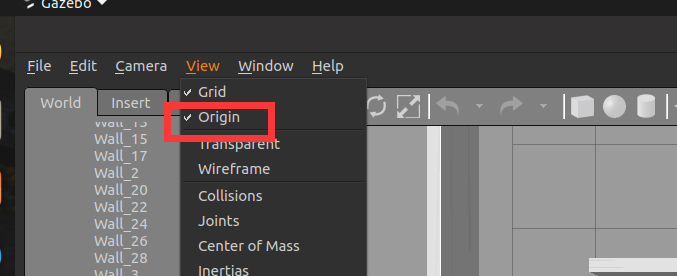本例中的截图如下所示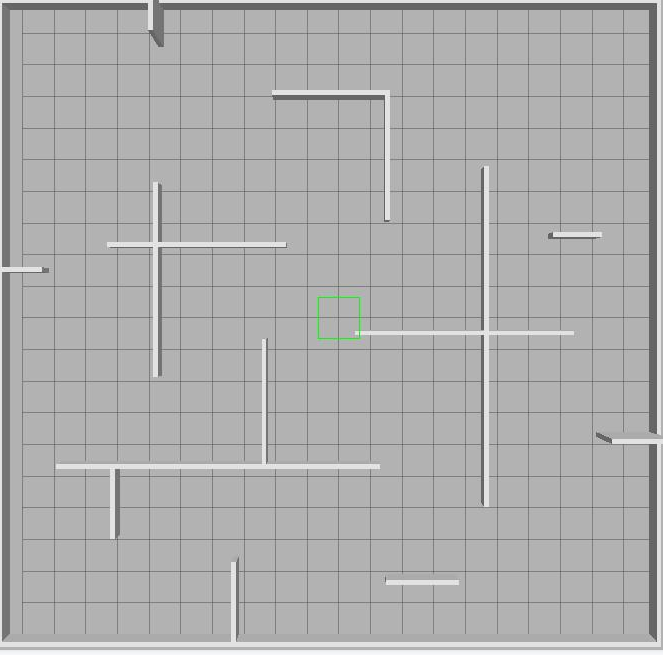在属性中可查看其像素值，也就是在MATLAB中以图片显示的地图的长度和宽度分别为666和656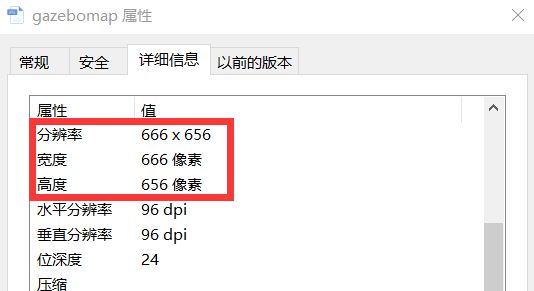（3）现在拿到了两幅地图的长度和宽度，但是在计算两幅地图的比例变换系数前，还要注意一个特别特别重要的问题，坐标系的变换问题，在MATLAB读取照片的时候，其坐标系的原点在左上角，而不是左下角，如下所示：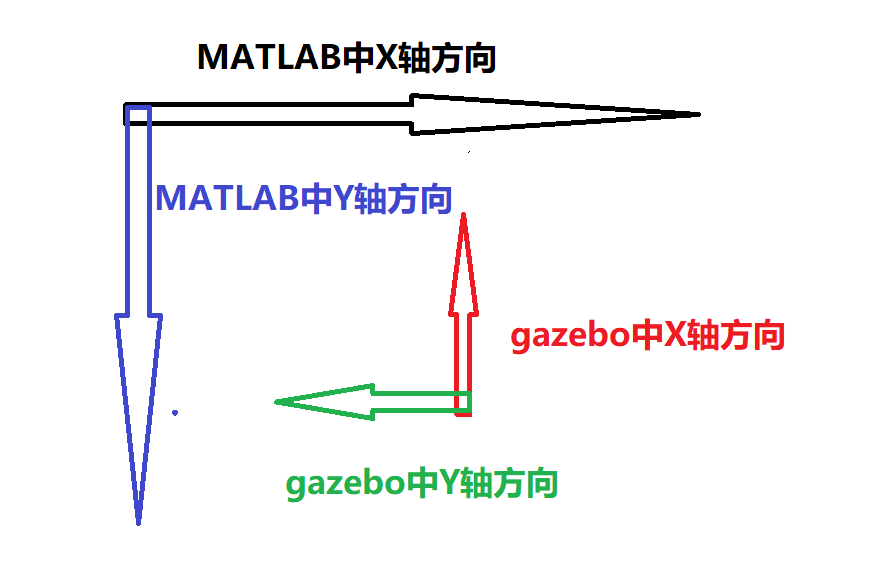也就是说我们得到的gazebo中X轴长度应该对应MALTAB中的Y轴的长度，gazebo中Y轴长度应该对应MALTAB中的X轴的长度，因此我们得到MATLAB中地图与gazebo中地图的比例为 X轴（以MATLAB为准）=666/20.35=32.72727，Y轴（以MATLAB为准）=656/19.85=33.04786
到这里我们就得到了两幅地图间的变换比例，也就是说在gazebo中小车沿着gazebo的的坐标系的X轴正方向移动1m，对应在MATLAB的地图中就是沿着Y轴的负方向移动了33.04786个像素点，同理，gazebo中小车沿着gazebo的的坐标系的Y轴正方向移动1m，对应在MATLAB的地图中就是沿着X轴的负方向移动了32.72727个像素点

二、调试获取两幅地图间的原点偏移量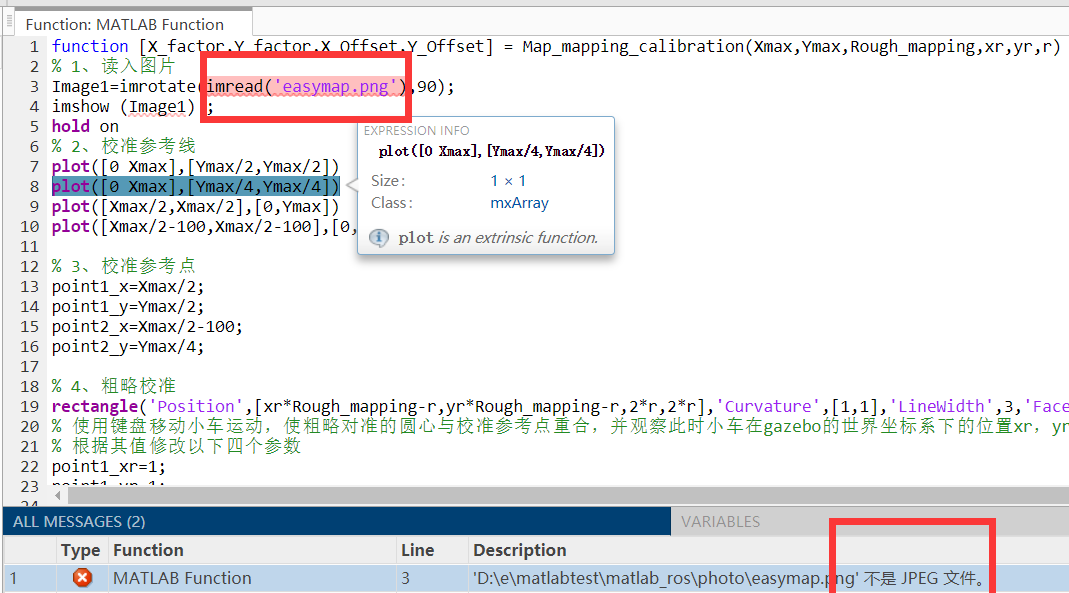Image1=im2gray(imread('gazebomap.jpg'));


在工作空间使用imshow函数将其显示出来，如下图所示：

    imshow(Image1);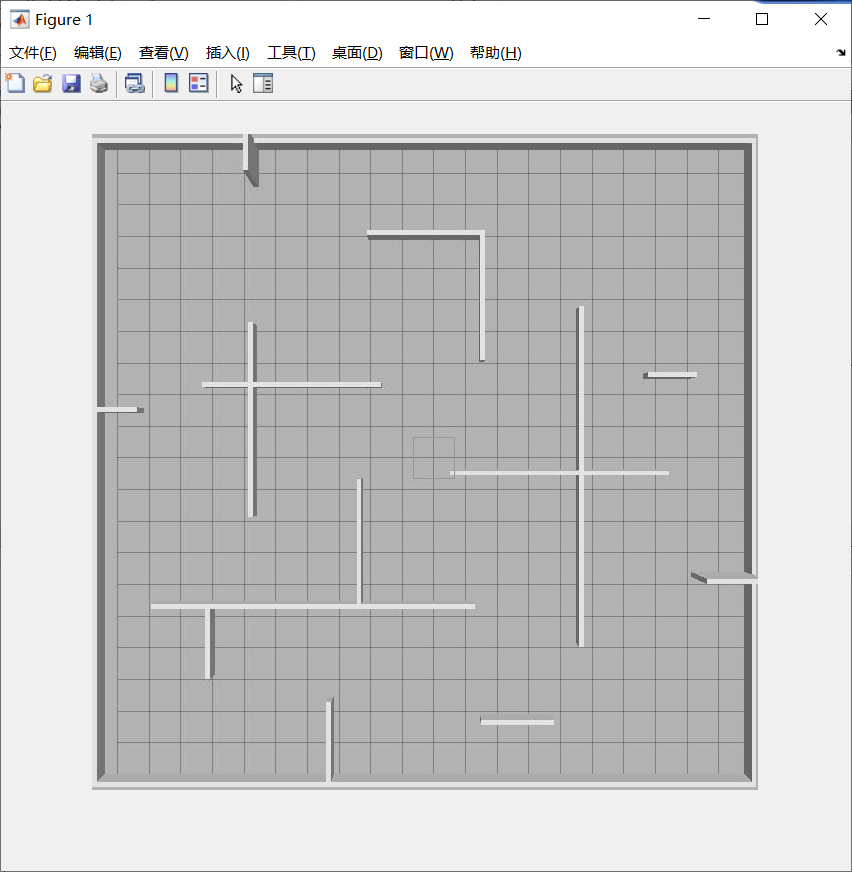（2）建立MATLAB与ROS的通讯，并通过subscribe模块订阅gazebo中发布的小车的位置信息，本部分内容在前面的文章中已经介绍过了，这里不再缀叙
（3）使用MATLAB Function模块来编写程序，在刚才MALTAB显示的图片基础上绘制小车的实时位置，其代码如下：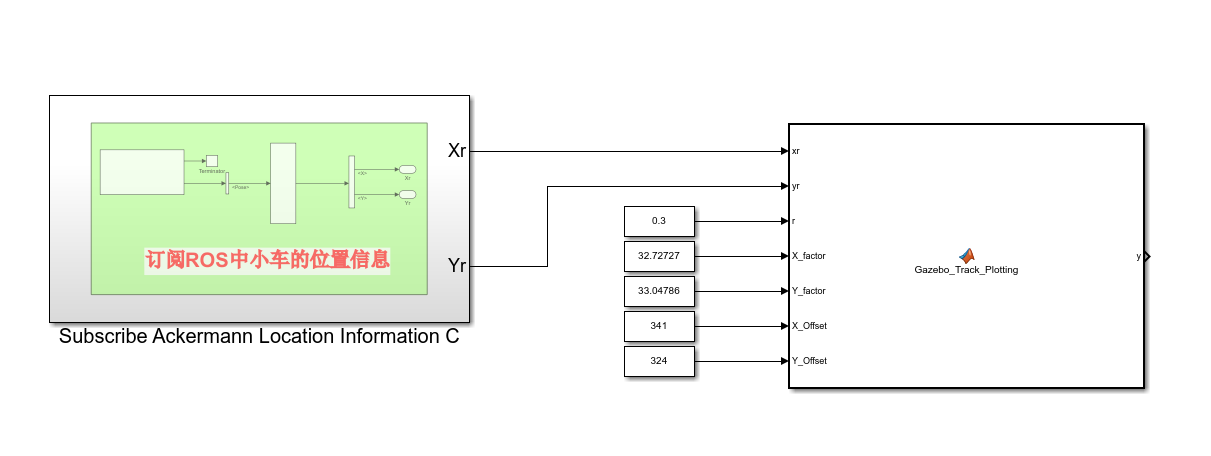function Gazebo_Track_Plotting(xr,yr,r,X_factor,Y_factor,X_Offset,Y_Offset)
hold on
%计算偏移量
rectangle('Position',[(-yr*X_factor+X_Offset)-r,(-xr*Y_factor+Y_Offset)-r,2*r,2*r],'Curvature',[1,1],'LineWidth',3,'FaceColor','y','EdgeColor','r'),axis equal
end


该函数的输入参数中xr，yr是订阅的小车的位置信息（是在gazebo的坐标系下的），X_factor和Y_factor是我们在第一部分计算出的两幅地图之间的变换比例关系，通过constant模块输入给该函数，即X_factor=32.72727，Y_factor=33.04786，X_Offset和Y_Offset就是本步我们要调试的两幅地图下原点的偏移量，由于本例中在gazebo地图中原点差不多在其地图的中点出，而在MATLAB中其原点在左上角，因此X_Offset和Y_Offset大概为图片像素值的一半，即X_Offset应该在666/2=333附近，Y_Offset应该在656/2=328附近，因此将这两个值作为初始值赋给该函数

由于matlab绘制单个单独的点不易观察，这里采用rectangle函数以该点为圆心绘制一个小圆的形式来代替了该点，r是该圆的半径

（4）在gazebo中选中仿真小车，在左侧将其X和Y坐标均设为0，这时，gazebo中小车就会移动至gazebo中地图的零点，如下所示：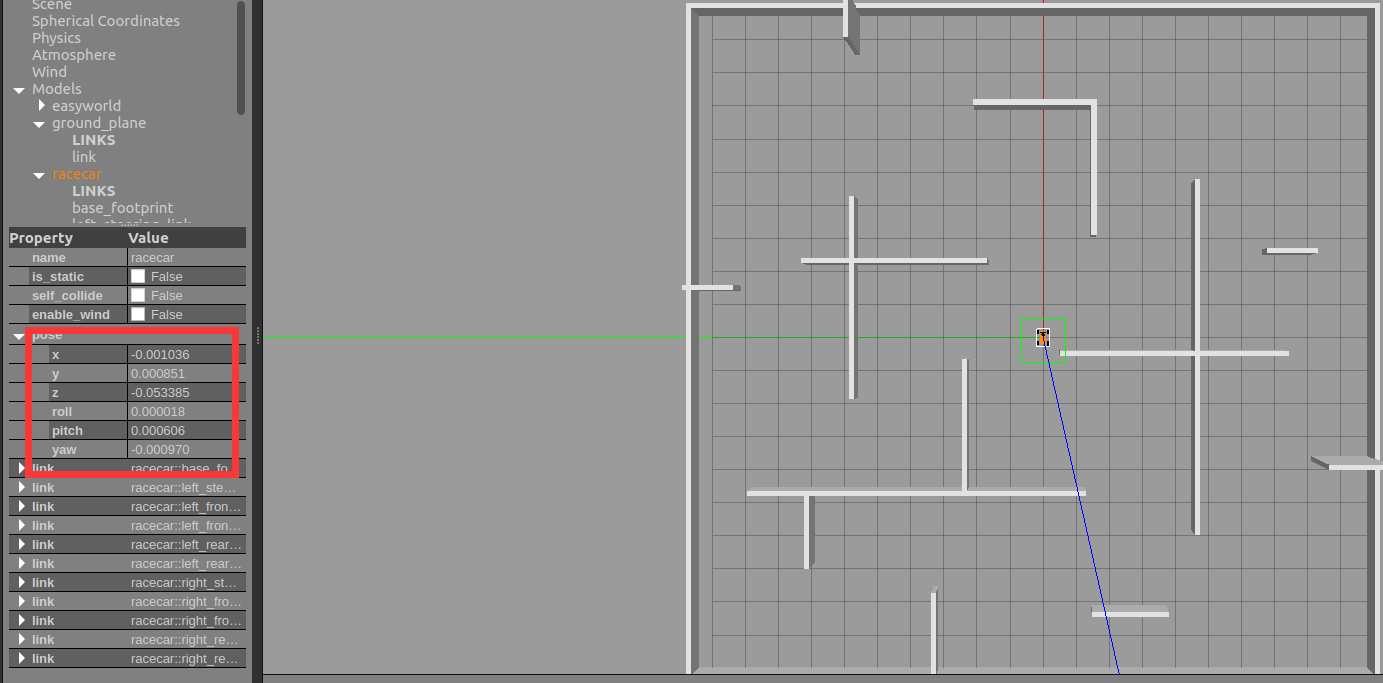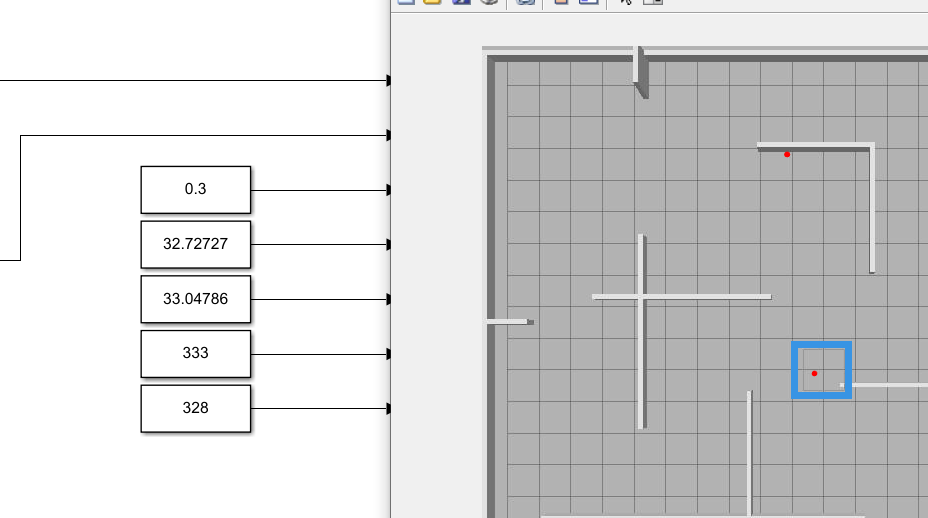可以发现其相对gazebo中小车的位置有点偏左和偏下，因此应该适当减少Y轴的偏移量，同时适当增加X轴的偏移量，调节传送给Gazebo_Track_Plotting函数的变量X_Offset和Y_Offset，再次观察红点的位置，如此循环直至红点显示的位置为gazebo中原点的位置，经过调节本例中的X_Offset应该改为341，Y_Offset应该改为324，如下图所示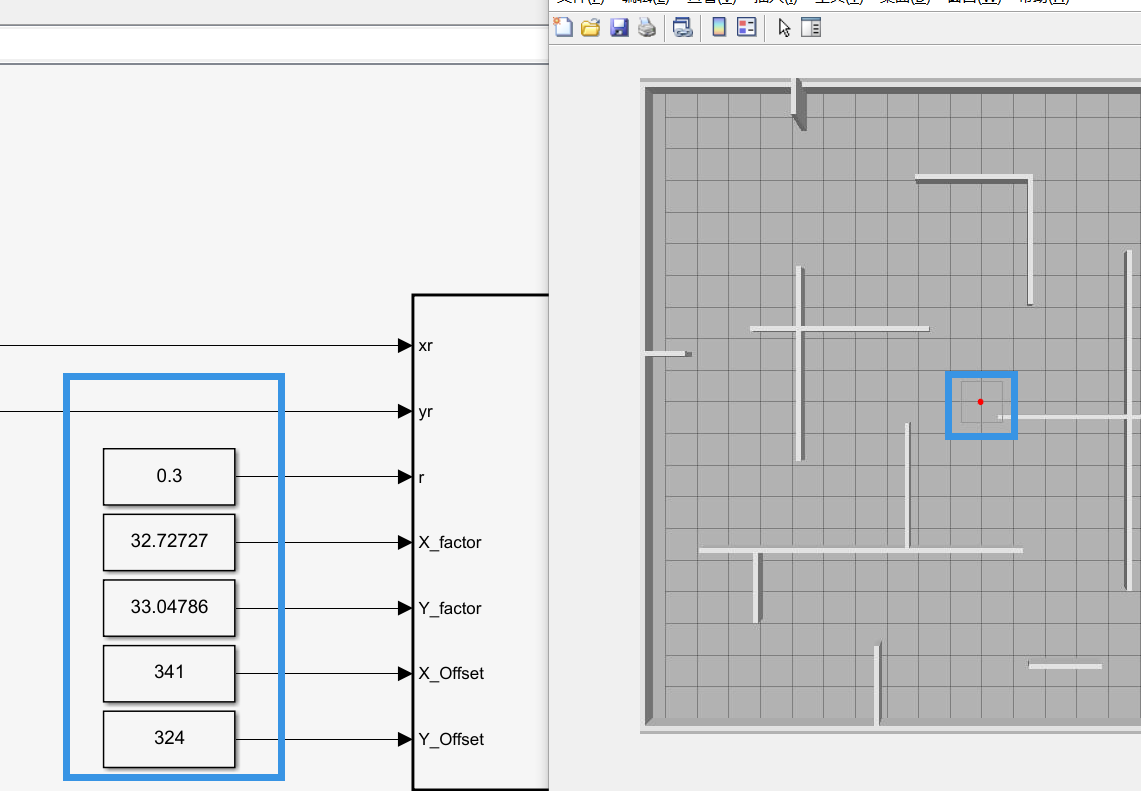到这里偏移量也就是找到了，同时，两个地图间的映射关系也就找到了

三、观察效果

控制gazebo中小车运动，观察MATLAB绘制的轨迹，如下所示：

在MATLAB中绘制Gazebo中小车的运行轨迹

虽然由于通讯的原因，轨迹的绘制有一定的延时性，但是大体上满足了预期的设想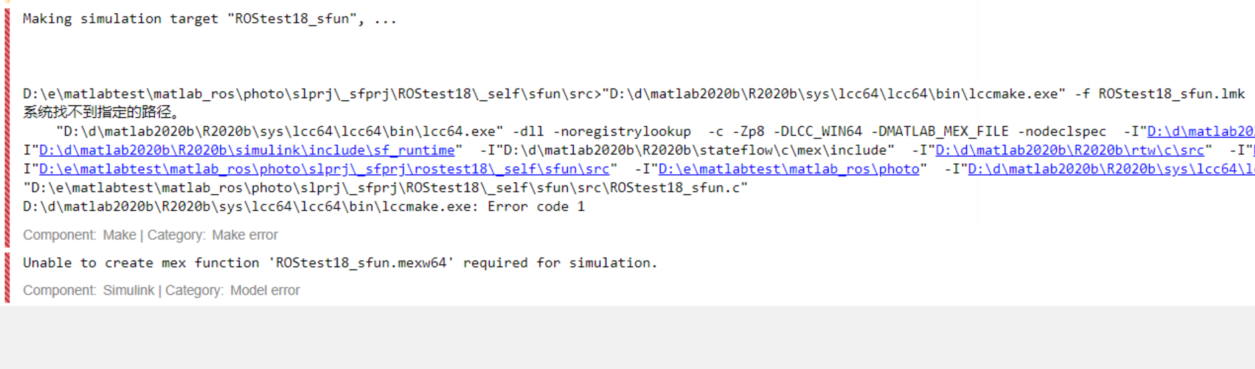展开全文• 室内定位出小车运动轨迹坐标(x,y)序列，怎么用matlab画小车运动轨迹，并保存为动态GIF格式

## s型无碳小车轨迹曲线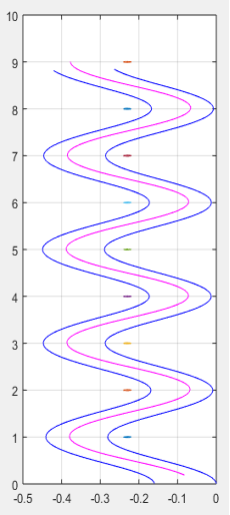## 单8型无碳小车轨迹曲线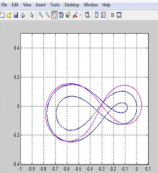## 双8字型无碳小车轨迹曲线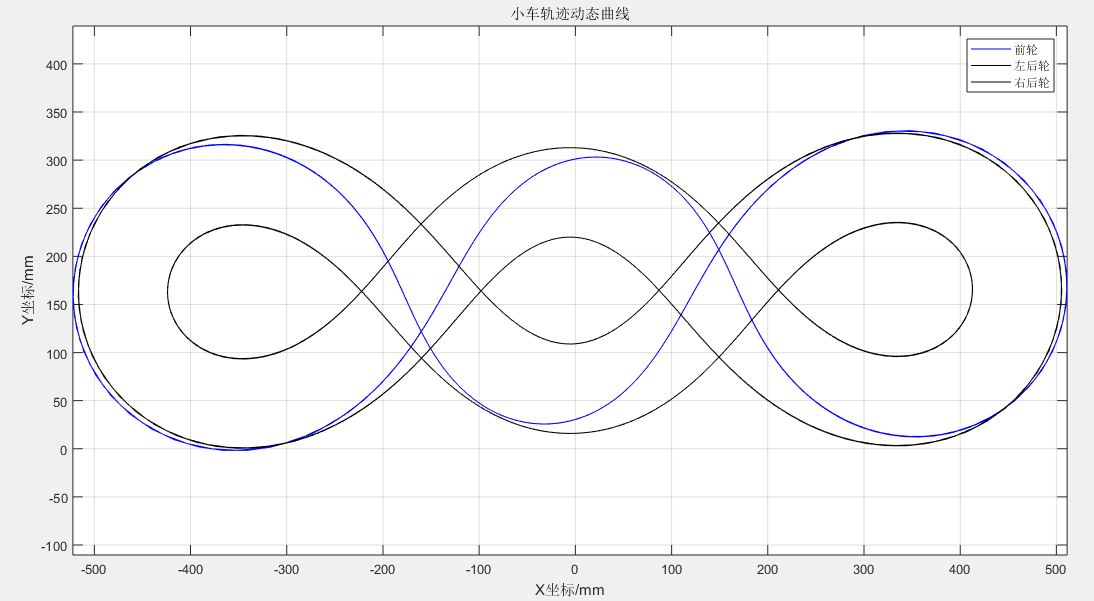展开全文• PID模糊小车直线控制，用MATLAB编写，运行后绘制小车运动轨迹
• 《基于无碳小车前轮运动关系的MATLAB运动轨迹仿真》由会员分享，可在线阅读，更多相关《基于无碳小车前轮运动关系的MATLAB运动轨迹仿真(4页珍藏版)》请在人人文库网上搜索。1、基于无碳小车前轮运动关系的MATLAB运动...
• 该课题为基于matlab运动汽车跟踪系统。读录视频，进行视频分帧，提取背景，框定运动目标。计算运动目标的数量，速度车道，车流密度等等信息带有人机交互界面框架，适合具备一定编程基础的人员学习。
• 从系统的稳定性、响应速度、超调量和稳态精度等各方面来考虑，、、的作 如下： 比例系数的作用是加快系统的响应速度，提高系统的调度。越大，系统的响应速度越快，系统的调节精度越高，但易产生超调，甚至会导致...增量式PID控制
• %% function [xyphi]=plant() clear all; close all; clc; %% l=2.9; %轴距 ts=0.001; t=0:ts:50; %定义时间序列 v(1:length(t))=5; %定义速度序列，匀速5m/s delta_deg=5*sin(0.5*t)+1*... % 前轮转角 单位为..
• 该课题为基于matlab运动汽车跟踪系统。读录视频，进行视频分帧，提取背景，框定运动目标。计算运动目标的数量，速度车道，车流密度等等信息带有人机交互界面框架，适合具备一定编程基础的人员学习。
• MATLAB检测运动车辆，包括轨迹，速度，车道，数量等，带GUI界面。
• 一、简介 1 卡尔曼滤波是什么 卡尔曼滤波适用于估计一个动态系统的最优状态。...假设我们手头有一辆DIY的移动小车。这辆车的外形是这样的： 这辆车可以在荒野移动，为了便于对它进行控制，需要知道它的位
• 为此,利用三维软件SoildWorks建立无碳小车的三维模型并对其结构进行分析,再利用Matlab软件进行仿真分析以实现各参数的最优化,根据分析结果对原有小车进行了结构上的创新设计,最后制造出小车实物。试验结果表明:该...
• 运用MATLAB使小车按着轨迹运行，进行跟踪标定。是MATLAB的实例运用。
• 前几天刚刚做了一个vrep小车仿真轨迹模拟的gui...在分析小车运动时，一个很重要的参数就是小车的三个轮子的运动速度，而小车的轮子属于joint类型 [查找文档](Enabling the B0-based remote API - client side (coppelilua
• MATLAB检测运动车辆，包括轨迹，速度，车道，数量等，带GUI界面。
• 用matlab绘制凸轮教程(详细)首先看一下理论轮廓线的方程式X (S0+S1)sinθ+ ecosθY S0+S1 cosθ+ esinθ式中，e为偏心距，S0 sqrt(r0^2-e^2)，r0为偏心圆半径%先设置凸轮的基本参数，偏心距离e，基圆半径rb，滚轮...
• 用matlab绘制凸轮教程(详细》由会员分享，可在线阅读，更多相关《用matlab绘制凸轮教程(详细(2页珍藏版)》请在人人文库网上搜索。1、下面我们来简单地介绍一下怎么样用matlab绘制凸轮的工作轮廓线主要涉及解析法...
• ## MATLAB 轮式机器人轨迹跟踪仿真

千次阅读 多人点赞 2021-10-24 13:01:21
控制目标是使机器人在二维平面中运动到指定位置，但由于轮式机器人不能进行平移运动，故其状态变量不能只取（x,y），应考虑车头朝向问题，所以小车的状态变量向量表示为： q=[x,y,θ]Tq=[x, y, \theta]^{T}q=[x,y,...开发语言
• 本文通过matlab，实现使用平面两自由度连杆，规定末端如下的8字形，反解关节速度、加速度，并带入动力学进行仿真。 理论 1,目标轨迹规划 本例中规划轨迹的8字形的方程如下所示： x=x0+Asin(aτ)y=y0+Bcos(bτ)&...
• 一、简介 1 卡尔曼滤波是什么 卡尔曼滤波适用于估计一个动态系统的最优状态。...假设我们手头有一辆DIY的移动小车。这辆车的外形是这样的： 这辆车可以在荒野移动，为了便于对它进行控制，需要知道.....

# 用matlab画小车运动轨迹matlab 订阅